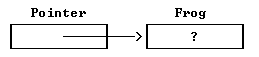XPL0 has two operators that return the address where a variable is stored. These are the two forms:           address VARIABLE         @VARIABLE           When either operator is written in front of a variable name, the value is no longer the contents of the variable, but the address in memory where the variable contents are stored. Because variable space is dynamically allocated, this address is not determined until a program executes. The two version work slightly differently   address = Always returns the 32-bit integer address of the variable. The variable can be an integer, a real, or  character, and it can be an array with a subscript. With any of the data types, the operator always returns a 32-bit integer address.   @ = The "@" operator works exactly the same for all variable types except a real. For a real, the operator returns the 32-bit memory address except that it is packed into the first half a 64-bit real. This allows the address to passed as in a real which allows it to work with real arrays   The "address" operation on a segment array (see: Segment Arrays) is not supported because segment arrays do not have simple 32-bit addresses.   "Address" is the reverse operation of subscripting an array name with zero. For example:           integer Frog, Pointer;         begin         Pointer:= address Frog;         if Pointer(0) = Frog then Text(0, "INVERSE OPERATORS");         . . ."INVERSE OPERATORS" is displayed despite the value initially contained in Frog because Pointer(0) and Frog both access the same memory location. The address operator can be used to solve a problem with multidimensional character arrays. Recall that a character array with a subscript always accesses a single byte. However, sometimes we want to access a 16-bit address. Look at this program:           char    S;         begin         S:= ["one", "two", "three", "four"];         ChOut(0, S(2,1));         S(1,1):= ^W;         Text(0, addr S(1,0));  \Caution: Text(0, S(1)); will not work         end;   When this runs, it displays:           htWo   Note that "addr S(1,0)" is used in the Text statement rather than "S(1)". This is because S(1) fetches a single byte rather than the entire word that holds the address of the string "tWo". Another solution would be to copy S into a temporary integer variable, for instance I, then I(1) would also fetch the desired address, but this is not as efficient.